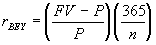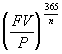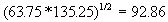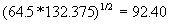Dr. P.V. Viswanath
 Home Bio Courses Research Economics/Finance on the Web Student Interest

 dFinancial Marketsd

# Financial Markets

### Money Market: The Market for Short Term Borrowing/ Lending

The money market refers to short-term marketable, liquid, low-risk debt securities. Capital markets include longer-term and riskier securities.

### Treasury Securities

T-bills represent promises of the government to the holder to pay a certain amount at a future date, usually within a year. Investors buy the bills at a discount from the government from the stated maturity value. The return to the holder is the difference between the stated value received at maturity and the price paid in the beginning.

T-bills are usually quoted using the bank discount yield., where FV is the face value of the bill, P is the price of the bill, and n is the number of days left to maturity on the bill. This is equal to the number of days left to maturity on the trade day less the one busines day allowed for payment, following the skip-day settlement convention.

The bond-equivalent yield on the bill is computed asThe effective annual yield on the bill is computed asminus 1.

Example:

Consider the following bills from the Wall Street Journal, quoted as of mid-afternoon, Oct. 12, 1999.

 Maturity Days to maturity Bid Asked Change Yield Oct 28 '99 16 4.40 4.32 -0.01 4.39 Nov 04 '99 23 4.44 4.36 -0.07 4.43

We can use the ask banker's discount quote to get the price.  The price, P, would satisfy the equation 0.0432 = [(100-P)/100](360/16).  Hence, P = 99.808 per \$100; so if the investor bought \$1m. worth of bills, the payoff at the end of the 16 days would be \$1m./0.99808 = \$1,001,923.70.  We can now use this price of 99.808 in the bond-equivalent yield formula.  We would find that [(100-99.808)/99.808](365/16) = 0.0439.  The effective annual yield can be computed as (1.0019237)(365/16) = 1.0448, or a rate of 4.48% per year.

You may also want to check these other links on Treasury Securities:

#### Stock Market Indexes

Using the following data, we will see how different kinds of market indexes are constructed:

 Stock Closing Price (Day 1) Closing Price (Day 2) No. of Shares Outstanding (millions) Value of Outstanding Stock (end of Day 1; billions) Value of Outstanding Stock (end of Day 2) Coca Cola 63.75 64.50 2480 158.1 159.96 Microsoft 135.25 132.375 1198 162.03 158.585

Return on Coca-Cola: 64.5/63.75 - 1 = 1.18%
Return on Microsoft: 132.375/135.25 - 1 = -2.13%

The Dow, which represents 30 large "blue-chip" corporations, was originally computed as the simple average of the prices of the stocks in the index. However, to ensure that the average did not change when there was a stock split, there were some adjustments to the weights, and hence to the divisor. The percentage change in the Dow measures the return on a portfolio that holds one share in each stock. The amount of money invested in each stock is equal to the price of that stock. .

The following example uses the data in the table above, and assumes that the weight of each stock is equal to one.

 Index value: Day 1 (63.75+135.25)/2 = 99.5 Index value: Day 2 (64.5+132.375)/2 = 98.4375 Percentage Price Change 98.4375/99.5 - 1 = -1.07%

If Coca Cola splits as of the end of day 2, then we need to adjust the divisor (currently 2), so that the value of the index does not change. This is necessary, if we presume that the stock split has not affected the "level" of the market. Assuming that the split simply halved the price of Coca Cola’s stock, the new divisor, D, must satisfy the equation (32.25+132.375)/D = 98.4375; i.e. D = 1.67238. As of January 29, 1998, the divisor was equal to 0.25

Although we have chosen to depict the index as the average price of a Dow stock, the index is meant to simply represent the "level" of the market. Consequently, we could multiply all index values by a constant, without changing the information in the index.

Note, however, that because of the nature of the construction of the Dow, the changes in the Dow Jones Index do not represent changes in the value of any portfolio. (See the article in the Economist, "A Poor Average," May 26, 2001, in the Investments section of the Media Articles page.)

S&P 500 Stock Index (market-value weighted average)

Represents a broad-based index of 500 stocks. The Index is effectively the total market value of all the stocks in the index on each day. Measures the return on a portfolio that invests an amount in each stock proportional to the market value of the outstanding shares.

 Index value: Day 1 158.1+162.03 = 320.13 Index value: Day 2 159.96+158.585 = 318.545 Percentage Price Change 318.545/320.13 = -0.495%

The Nasdaq Composite Index is another market-value weighted index.

Equally weighted indexes

Measures the value of a portfolio that invests an equal amount in each stock at each point in time. The percentage change in the index does not correspond to the return on any buy-and-hold portfolio. For example, on day 1, to achieve the goal of investing an equal number of dollars in each stock, if we were to invest \$50 in each stock, we would have to buy 50/63.75 = 0.7843 units of stock 1 and 50/135.25 = 0.3697 units of stock 2. The total portfolio values are given below

 Index value: Day 1 0.7843(63.75) + .3697(135.25) = 100 Index value: Day 2 0.7843(64.5) + .3697(132.375) = 99.526 Percentage Price Change 99.526/100 - 1 = -0.47%

This percentage price change is equal to the simple average of the returns on the two stocks, (1.18 + (-2.13))/2 = -0.47. Hence the new index value would be 100(1-0.0047) or 99.526.

On day 2, the same number of shares in each stock would no longer be an equally-weighted portfolio, since the Microsoft shares would be worth 0.3697(132.375) or \$48.94, while the Coca-Cola shares would be worth 0.7843(64.5) = \$50.59. Hence the next index value is computed by taking the simple average of the returns on the two stocks from day 2 to day 3 and grossing the index by that amount. For example, if Microsoft traded at 133 on day 3 and Coca-Cola at 65, their respective returns would be 0.47% (133/132.375) and 0.78% (65/64.5 - 1); the simple average is 0.63%, and 99.526(1.0063) = 100.1491, which would be the next index value.

Value Line Geometric Average Index

The index is computed as the geometric average of the prices of the stocks in the index. The percentage change in the index is equal to the equally weighted geometric average of the performance of about 1700 firms. Does not correspond to the return on any portfolio. Downward biased estimate of the return on an equally-weighted portfolio.

 Index value: Day 1Index value: Day 2Percentage Price Change 92.4/92.86 - 1 = -0.49%